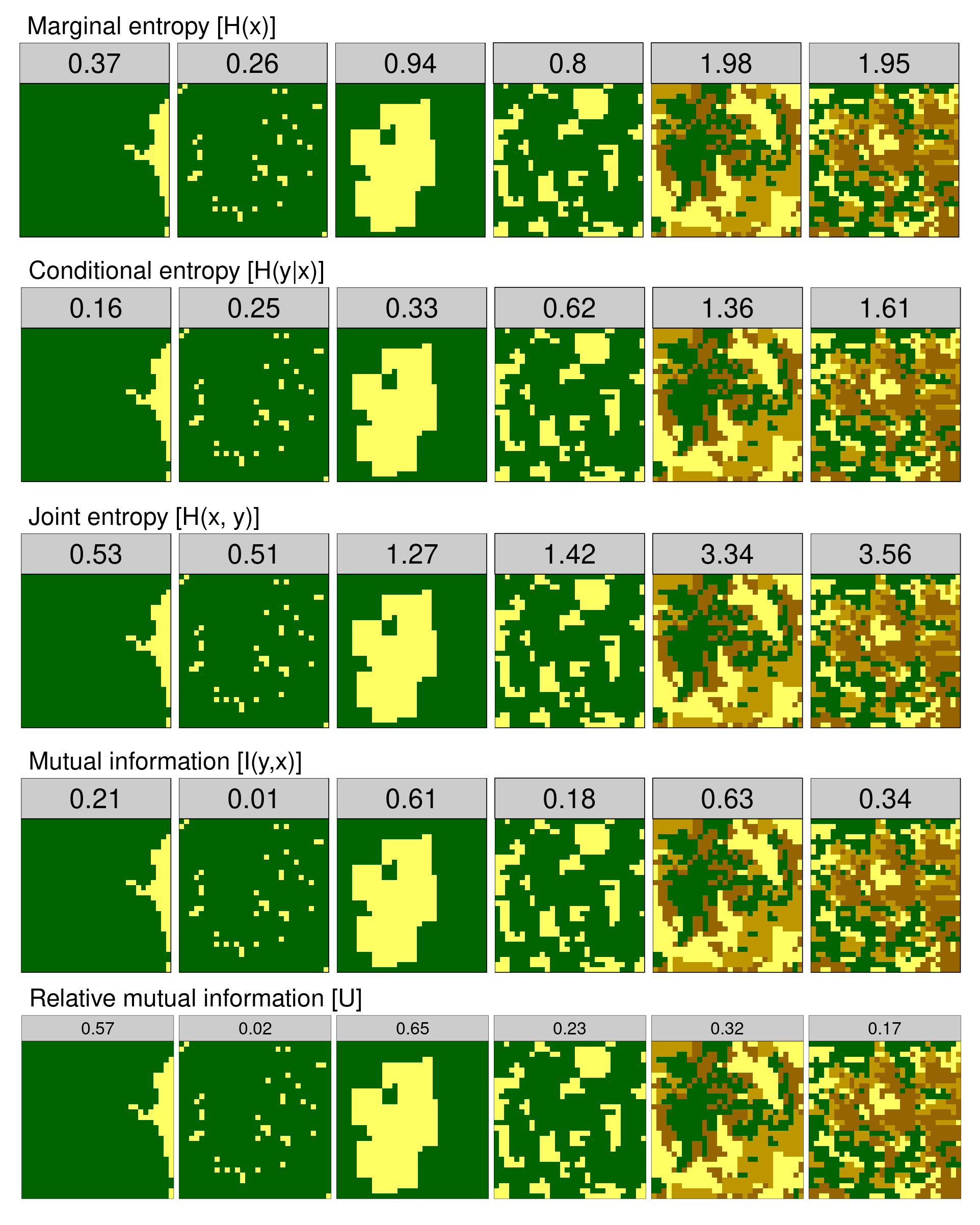One of the reason to start landscapemetrics was also to have a collection of metrics that are not included in FRAGSTATS. This vignette will highlight them and provide references for further reading on them.

## Information theory-based framework for the analysis of landscape patterns

Nowosad J., TF Stepinski. 2019. Information theory as a consistent framework for quantification and classification of landscape patterns. https://doi.org/10.1007/s10980-019-00830-x

Information-theoretical framework can be applied to derive four metrics of landscape complexity: Marginal entropy [H(x)], Conditional entropy [H(y|x)], Joint entropy [H(x, y)], and Mutual information [I(y,x)].

All of these metrics are implemented in landscapemetrics:

• lsm_l_ent - Marginal entropy [H(x)]. It represents a diversity (thematic complexity, composition) of spatial categories. It is calculated as the entropy of the marginal distribution.
• lsm_l_condent - Conditional entropy [H(y|x)]. It represents a configurational complexity (geometric intricacy) of a spatial pattern. If the value of conditional entropy is small, cells of one category are predominantly adjacent to only one category of cells. On the other hand, the high value of conditional entropy shows that cells of one category are adjacent to cells of many different categories.
• lsm_l_joinent - Joint entropy [H(x, y)]. It is an overall spatio-thematic complexity metric. It represents the uncertainty in determining a category of the focus cell and the category of the adjacent cell. In other words, it measures diversity of values in a co-occurrence matrix – the smaller the diversity, the larger the value of joint entropy.
• lsm_l_mutinf - Mutual information [I(y,x)]. It quantifies the information that one random variable (x) provides about another random variable (y). It tells how much easier is to predict a category of an adjacent cell if the category of the focus cell is known. Mutual information disambiguates landscape pattern types characterized by the same value of overall complexity.
• lsm_l_relmutinf - Relative mutual information. Due to the spatial autocorrelation, the value of mutual information tends to grow with a diversity of the landscape (marginal entropy). To adjust this tendency, it is possible to calculate relative mutual information by dividing the mutual information by the marginal entropy. Relative mutual information always has a range between 0 and 1 and can be used to compare spatial data with different number and distribution of categories.For more information read the Information theory provides a consistent framework for the analysis of spatial patterns blog post.